## *EXPANSION

Keyword type: model definition, material

This option is used to define the thermal expansion coefficients of a material. They are interpreted as total expansion coefficients with respect to a reference temperature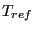, i.e. the thermal strain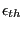of a material at a final temperature T and with initial temperature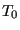is determined by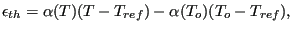(624)

where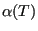is the thermal coefficient at a temperature T. There are two optional parameters TYPE and ZERO. Default for TYPE is TYPE=ISO, other values are TYPE=ORTHO for orthotropic materials and TYPE=ANISO for anisotropic materials. All constants may be temperature dependent. The parameter ZERO is used to determine the reference temperature, default is 0.

First line:

• *EXPANSION
• Enter the TYPE and ZERO parameters and their values, if needed

Following line for TYPE=ISO:

•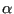.
• Temperature.
Repeat this line if needed to define complete temperature dependence.

Following line for TYPE=ORTHO:

•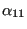.
•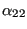.
•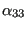.
• Temperature.
Repeat this line if needed to define complete temperature dependence.

Following line for TYPE=ANISO:

•.
•.
•.
•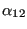.
•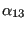.
•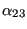.
• Temperature.
Repeat this line if needed to define complete temperature dependence.

Example:

*EXPANSION,ZERO=273.
12.E-6,373.
20.E-6,573.


tells you that the thermal strain in a body made of this material is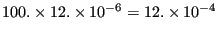if heated from T=273 to T=373, and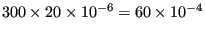if heated from T=273 to T=573.

Example files: beamt, beamt2.